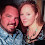## Monday, February 24, 2014

### Judge and Jury Numerology | The Language of Numbers

The word 'Judge' has the following numerology.
• Judge = 10+21+4+7+5 = 47
• Obey = 47 (Simple English, like above)
• Authority = 47 (Pythagorean)
The word 'Jury' has the following numerology.
• Jury = 10+21+18+25 = 74
Thus, the numerology of the words 'Judge' and 'Jury' are the inverse of each other, respectively; 47:74.  They also both breakdown to a numerology of 11:11.
• 47 = 4+7 = 11
• 74 = 7+4 = 11
• Judge (47)+Jury (74) = 121
• 11x11 = 121
• Judge and Jury both have a numerology of 11...
A judge and jury serve the purpose of helping uphold the law.
• Law = 3+1+5 = 9 (Pythagorean)
• Law = 12+1+23 = 36 = 3+6 = 9 (Standard)
• When you sum 1 through 36, you get 666
• three-six... 666?
And of course there are lawyers.
• Lawyer = 3+1+5+7+5+9 = 30 = 3+0 = 3 (Pythagorean)
• Lawyer = 12+1+23+25+5+18 = 84 = 8+4 = 12 = 1+2 = 3 (Standard)
• 8/4 is President Obama's birthday, the Constitutional Lawyer
• United States of America = 84 (Pythagorean)
• Obama = 84 (Jewish Gematria)
In the time of Jesus Christ, he was crucified on the cross, for going against Roman Law.
• Jesus = 10+5+19+21+19 = 74
• Cross = 3+18+15+19+19 = 74
• Christ = 3+8+18+9+19+20 = 77
• Court = 3+15+21+18+20 = 77
Jesus was crucified at the age of 33, after performing 33 miracles.  In Genesis, his angelic form is referred to as Michael.  Michael also has a numerology of 33.
• Michael = 4+9+3+8+1+5+3 = 33
The 33rd State is Oregon.  It has an abbreviation of OR.
• OR = 15+18 = 33
• Oregon = 15+18+5+7+15+14 = 74
The United States Declaration of Independence is credited with a 7/4 birthday, on July 4, 1776.  In the book of Revelation, the 22 part finale to the Bible, the numbers 7 and 4 are the most often used of any numbers, by a large margin.
It leaves us with this question:  What does it all mean?

1.2.